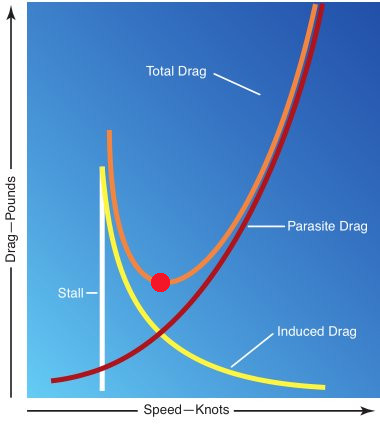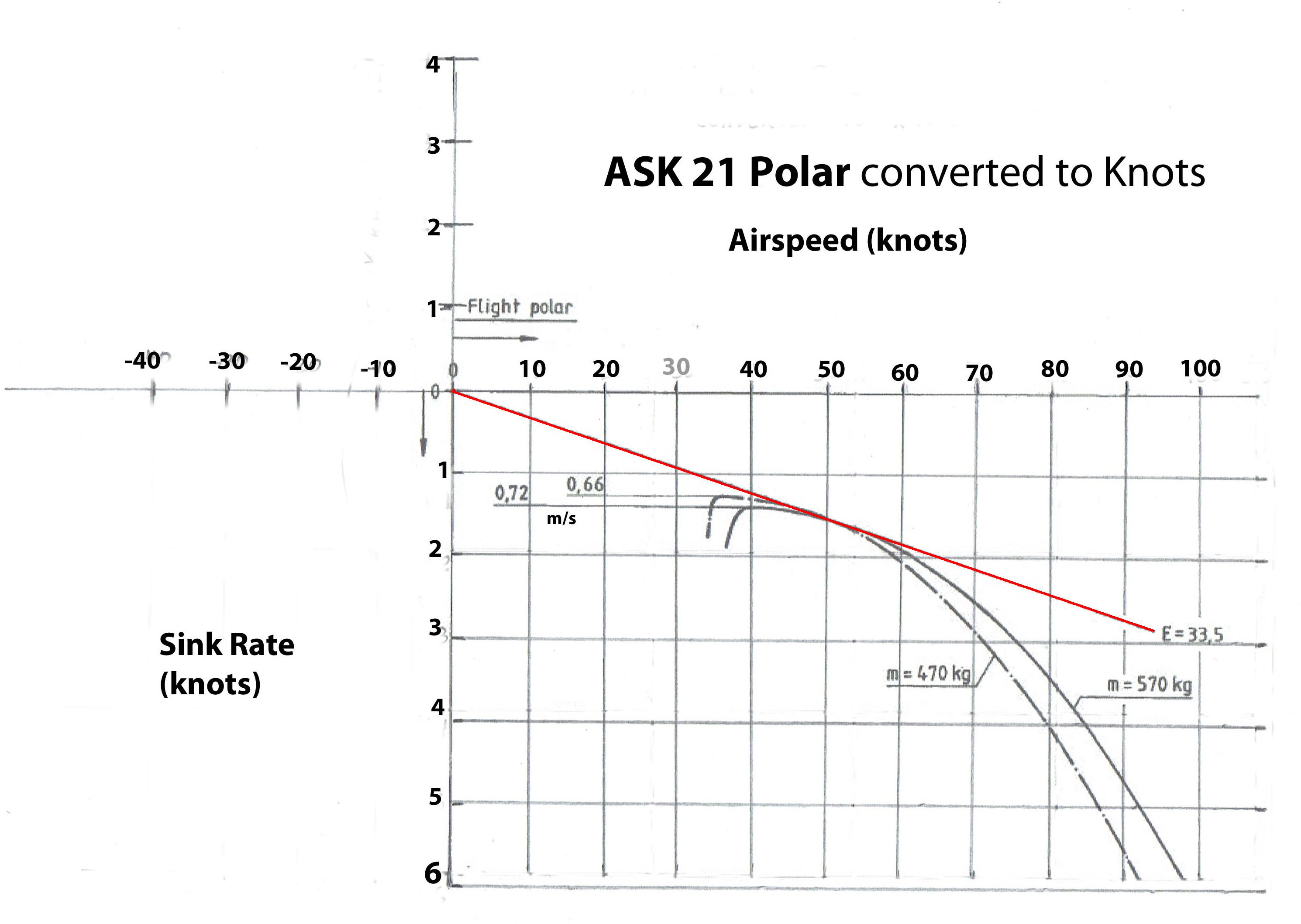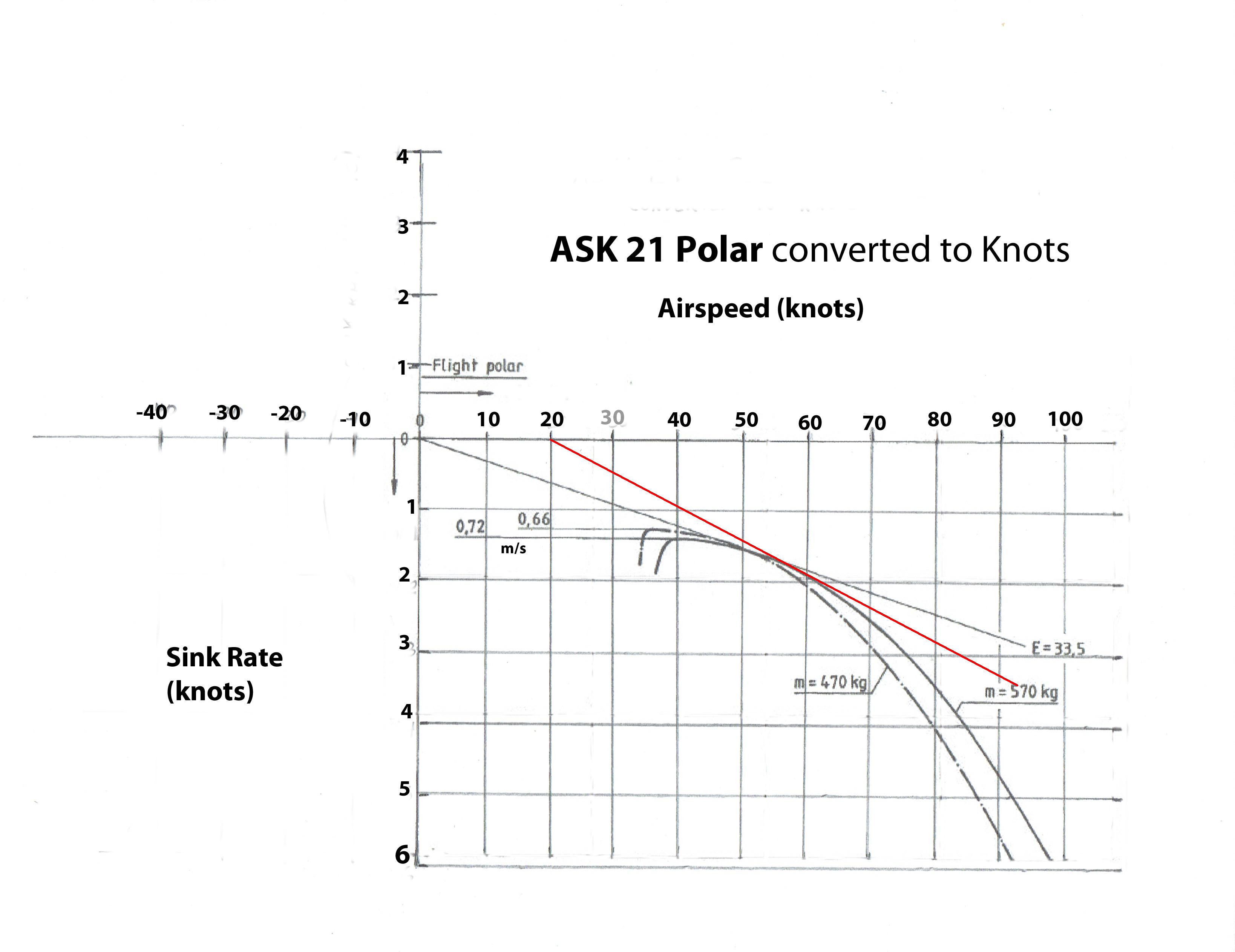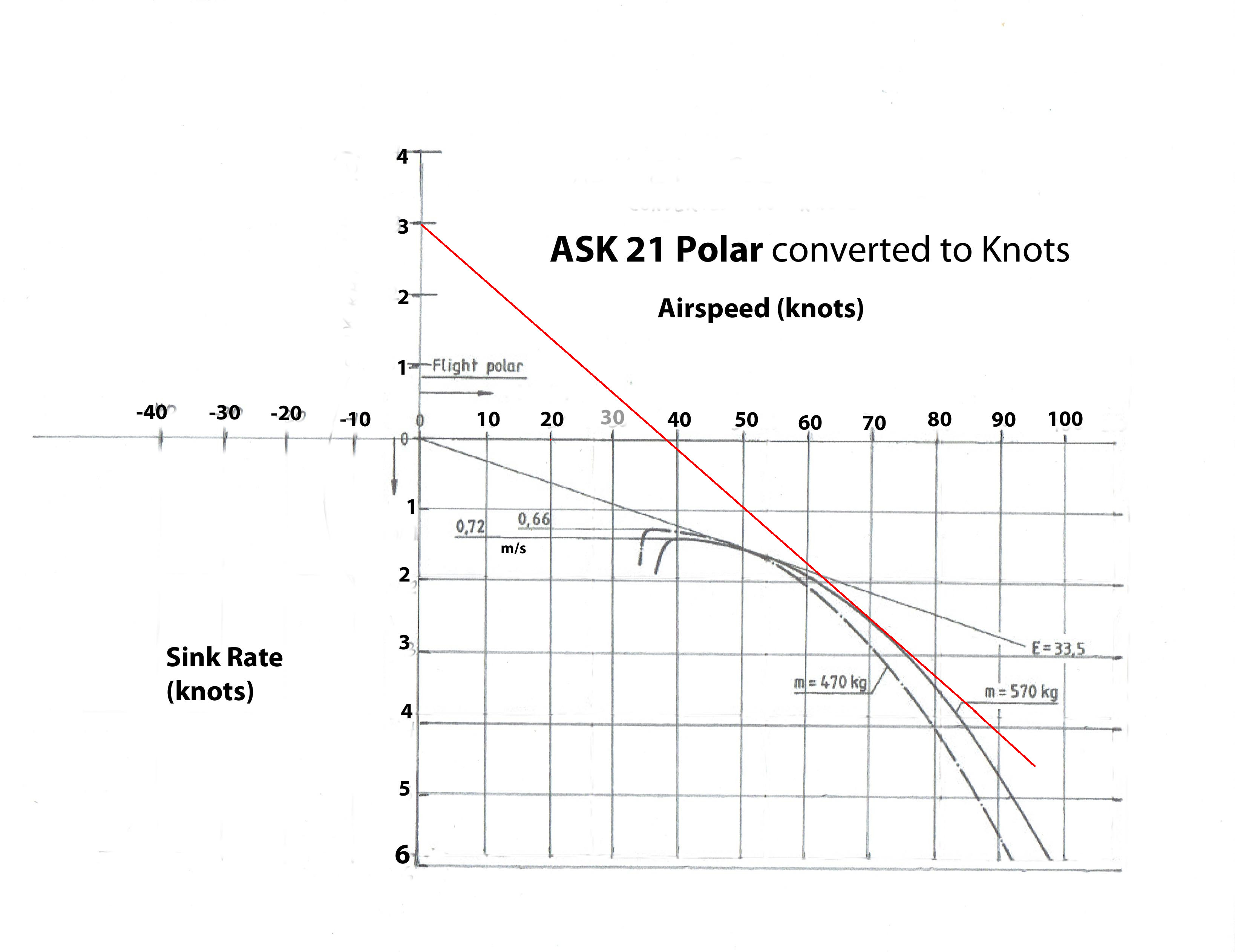# Nashville CFI

### LDmax (Best Glide)

The best glide speed of an aircraft is the speed at which it will travel the most forward distance for the least amount of altitude lost. In many high performances gliders, this can be as much as 50 feet forward for every 1 foot of altitude lost (a 50:1 glide ratio). In many training gliders, it's closer to 30:1.

In every case, the best glide ratio is found at the point where the total drag of the glider is the least, as shown in the drag graph below. Total drag is represented by the orange line, and the red dot at the bottom of it represents the airspeed at which the aircraft has the lowest drag possible. Come straight down from the red dot to find the airspeed at which this occurs, and that will be your best glide speed.### Flight Polar

A "polar" is a performance graph from the POH of a glider which shows the performance of the glider (or sink rate) at various airspeeds. Here is one from the Schleicher ASK21 which has been converted to knots. Once again, hat tip to CFI-G Tom Fleming for creating this graph!

The best glide speed is found by starting at the 0,0 point, and drawing a line tangent to the sink rate line. From the point of the tangent that intersects the sink rate line, go straight up to determine the airspeed for best glide. We find at gross weight this to be 49 knots for the ASK21. See below.But what if we had a 20 knot headwind? We know intuitively we need to speed up to get the best glide speed, but how much? The polar shows us that, too.

Simply slide your starting point to the right and start your tangent line from the amount of headwind, and then go up to determine the best glide airspeed. In this case, we find it is almost 60 knots.### Flight Polar in Sink

One more possibility is that we find ourselves in a lot of sink (story of my life). Should we fly around in sink at 49 knots or something else? The polar will tell us.

Start your tangent line at the level of sink on the Y-axis of the chart (3 knots here). Find where the line is tangent to (intersects) the polar line, and then come straight up from that point to find the proper airspeed for 3 knots of sink. We find that it is 70 knots.